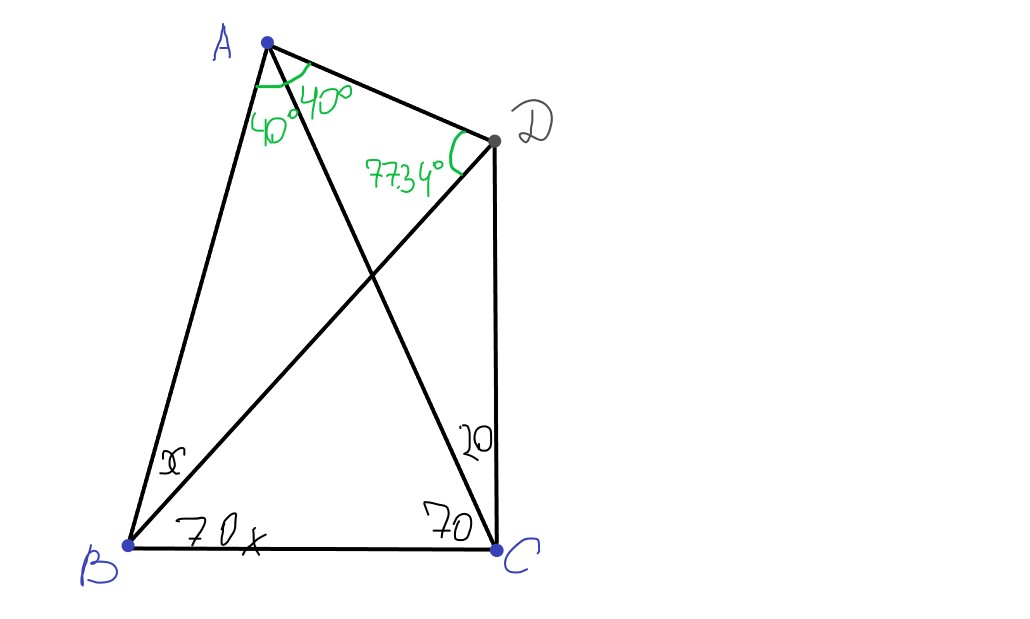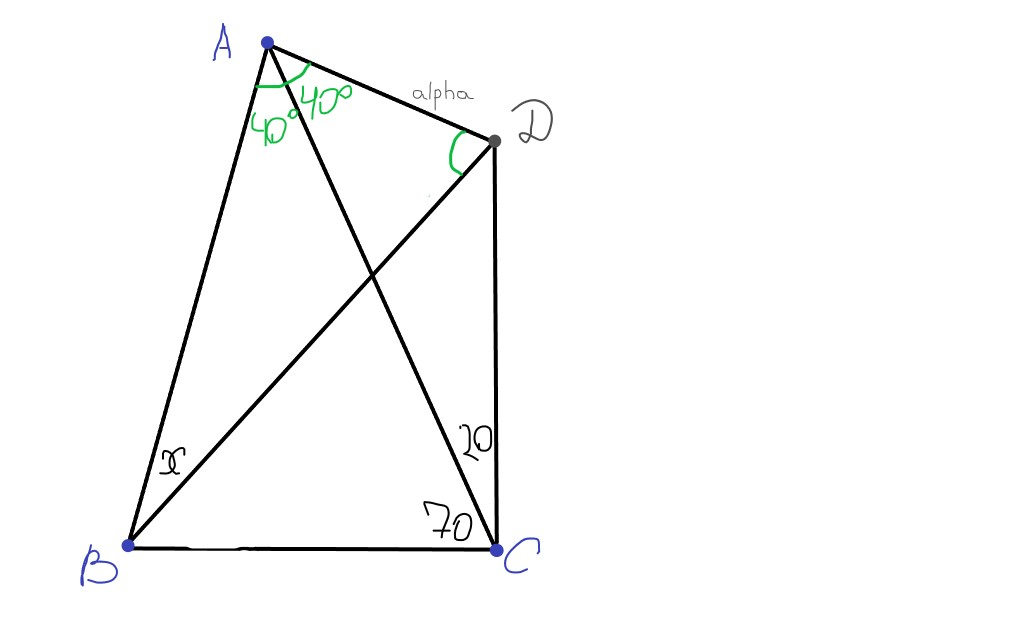# Let quadrilateral ABCD satisfy angle BAC= angle CAD=2 angle ACD=40^circ and angle ACB=70^circ. Find angle ADB.Ximena Skinner 2022-07-15 Answered
Let quadrilateral ABCD satisfy $\mathrm{\angle }BAC=\mathrm{\angle }CAD=2\phantom{\rule{thinmathspace}{0ex}}\mathrm{\angle }ACD={40}^{\circ }$ and $\mathrm{\angle }ACB={70}^{\circ }$. Find $\mathrm{\angle }ADB$.
You can still ask an expert for help

• Live experts 24/7
• Questions are typically answered in as fast as 30 minutes
• Personalized clear answers

Solve your problem for the price of one coffee

• Math expert for every subject
• Pay only if we can solve itDalton Lester
Step 1
$\approx 77,{34}^{\circ }$, soStep 2
$\frac{\mathrm{sin}80}{\mathrm{sin}40}\frac{\mathrm{sin}\left(70-x\right)}{\mathrm{sin}x}\frac{\mathrm{sin}20}{\mathrm{sin}90}=1$
After some manipulation we get $\mathrm{cot}x=\mathrm{tan}20+\frac{2}{\mathrm{cos}10}\phantom{\rule{thickmathspace}{0ex}}⟹\phantom{\rule{thickmathspace}{0ex}}x=...$
###### Not exactly what you’re looking for?Joel FrenchSince $\mathrm{\angle }ACB=\mathrm{\angle }ABC={70}^{\circ }$, the triangle ABC is isosceles and $\phantom{\rule{thickmathspace}{0ex}}\overline{AB}=\overline{AC}$.
By applying the law of sines to the triangle ACD, we get that:
$\overline{AD}=\overline{AC}\cdot \frac{\mathrm{sin}\mathrm{\angle }ACD}{\mathrm{sin}\mathrm{\angle }ADC}=\overline{AC}\cdot \frac{\mathrm{sin}{20}^{\circ }}{\mathrm{sin}{120}^{\circ }}=\frac{2\overline{AC}\mathrm{sin}{20}^{\circ }}{\sqrt{3}}\phantom{\rule{thickmathspace}{0ex}}.$
And, by applying the law of sines to the triangle ABD, we get that:
$\overline{AD}\mathrm{sin}\mathrm{\angle }ADB=\overline{AB}\mathrm{sin}\mathrm{\angle }ABD\phantom{\rule{thickmathspace}{0ex}}.\phantom{\rule{1em}{0ex}}\left(\ast \right)$
Let $\phantom{\rule{thickmathspace}{0ex}}\alpha =\mathrm{\angle }ADB\phantom{\rule{thickmathspace}{0ex}}.$.
Step 2
Since $\phantom{\rule{thickmathspace}{0ex}}\overline{AD}=\frac{2\overline{AC}\mathrm{sin}{20}^{\circ }}{\sqrt{3}}\phantom{\rule{thickmathspace}{0ex}}$, $\phantom{\rule{thickmathspace}{0ex}}\overline{AB}=\overline{AC}\phantom{\rule{thickmathspace}{0ex}}$ and $\phantom{\rule{thickmathspace}{0ex}}\mathrm{\angle }ABD={100}^{\circ }-\alpha \phantom{\rule{thickmathspace}{0ex}},\phantom{\rule{thickmathspace}{0ex}}$, the equality (∗) turns into:
$\frac{2\overline{AC}\mathrm{sin}{20}^{\circ }\mathrm{sin}\alpha }{\sqrt{3}}=\overline{AC}\mathrm{sin}\left({100}^{\circ }-\alpha \right)\phantom{\rule{thickmathspace}{0ex}},$
$2\mathrm{sin}{20}^{\circ }\mathrm{sin}\alpha =\sqrt{3}\mathrm{sin}\left({90}^{\circ }+{10}^{\circ }-\alpha \right)\phantom{\rule{thickmathspace}{0ex}},$
$4\mathrm{sin}{10}^{\circ }\mathrm{cos}{10}^{\circ }\mathrm{sin}\alpha =\sqrt{3}\mathrm{cos}\left({10}^{\circ }-\alpha \right)\phantom{\rule{thickmathspace}{0ex}},$
$4\mathrm{sin}{10}^{\circ }\mathrm{cos}{10}^{\circ }\mathrm{sin}\alpha =\sqrt{3}\left(\mathrm{cos}{10}^{\circ }\mathrm{cos}\alpha +\mathrm{sin}{10}^{\circ }\mathrm{sin}\alpha \right)\phantom{\rule{thickmathspace}{0ex}},$
$4\mathrm{sin}{10}^{\circ }\mathrm{sin}\alpha =\sqrt{3}\left(\mathrm{cos}\alpha +\mathrm{tan}{10}^{\circ }\mathrm{sin}\alpha \right)\phantom{\rule{thickmathspace}{0ex}},$
$\left(4\mathrm{sin}{10}^{\circ }-\sqrt{3}\mathrm{tan}{10}^{\circ }\right)\mathrm{sin}\alpha =\sqrt{3}\mathrm{cos}\alpha \phantom{\rule{thickmathspace}{0ex}},$
$\mathrm{tan}\alpha =\frac{\sqrt{3}}{4\mathrm{sin}{10}^{\circ }-\sqrt{3}\mathrm{tan}{10}^{\circ }}\phantom{\rule{thickmathspace}{0ex}}.$
Hence, $\mathrm{\angle }ADB=\alpha =\mathrm{arctan}\left(\frac{\sqrt{3}}{4\mathrm{sin}{10}^{\circ }-\sqrt{3}\mathrm{tan}{10}^{\circ }}\right)\simeq \phantom{\rule{0ex}{0ex}}\simeq 77,{3361794}^{\circ }.$